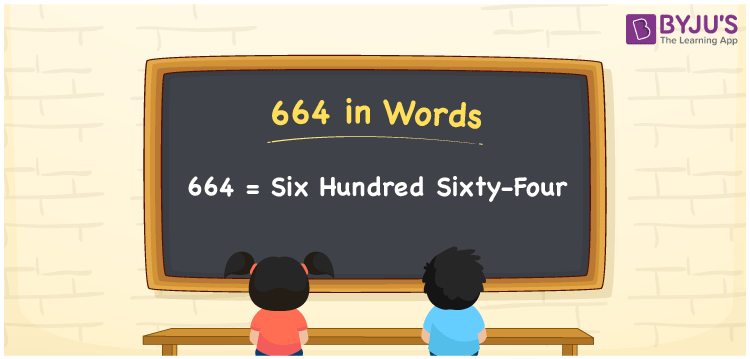# 664 in Words

We can write 664 in words as Six hundred sixty-four. If you designed 664 sarees in a year, then you can say, “I designed Six hundred sixty-four sarees in a year”. Here, Six hundred sixty-four is known as the word form of 664. Also, we can say that 664 is a cardinal number since it defines a specific amount or quantity.

 664 in words Six hundred sixty-four Six hundred sixty-four in Numbers 664

## 664 in English Words

We generally use the English alphabet to express numbers in words. So, we spell 664 in English words as “Six hundred sixty-four”.## How to Write 664 in Words?

In this section, you will learn how to write the number 664 in words with the help of a place value chart. The below table shows the place values for all the digits of 664.

 Hundreds Tens Ones 6 6 4

Here, ones = 4, tens = 6, hundreds = 6

So, 6 × Hundred + 6 × Ten + 4 × One

= 6 × 100 + 6 × 10 + 4 × 1

= 600 + 60 + 4

= Six hundred + Sixty + Four

= Six hundred sixty-four

Therefore, 664 in words is written as Six hundred sixty-four.

Learn in detail about place value here.

664 is a natural number that precedes 665 and succeeds 663.

664 in words – Six hundred sixty-four

Is 664 an odd number? – No

Is 664 an even number? – Yes

Is 664 a prime number? – No

Is 664 a composite number? – Yes

Is 664 a perfect square number? – No

Is 664 a perfect cube number? – No

## Frequently Asked Questions on 664 in Words

Q1

### What is 664 in words?

Six hundred sixty-four represents the number 664 in words.
Q2

### Write the number name of 664.

We can write the number name of 664 as Six hundred sixty-four.
Q3

### How to write Rs. 664 in words on a cheque?

On a cheque, we generally express Rs. 664 in words as “Six hundred sixty-four rupees only”.